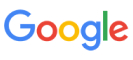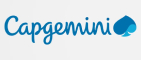New update is available. Click here to update.
Topics

# Ninja and Numbers

Moderate0/80
Average time to solve is 25m## Problem statement

Ninja has a number ‘A’ containing ‘B’ digits. ‘A’ can be represented by a string ‘S’ where ‘S[i]’ denotes the ‘ith’ digit of ‘A’. You are also given an integer ‘K’.

Ninja thinks that a number is stable if the following condition is satisfied:

For every ‘ith’ digit where (0 <= ‘i’ <= ‘B-1’) ‘S[i] = S[i%K]’. Here, ‘X%Y’ represents the modulo operations. The remainder when ‘X’ is divided by ‘Y’.

Your task is to find the smallest number which is stable and whose value is greater than or equal to ‘A’. Zero-based indexing is used everywhere.

Example :
``````‘B’ = 4, ‘S’ = “4321”, ‘K’ = 3.
The given number is not stable as ‘S’ is not the same as ‘S’ but 3%3 = 0 same as 0%3. ‘S = 1’ and ‘S = 4’.  But the number “4324” is stable. As, for all ‘i’, ‘S[i]’ = ‘S[i%K]’ and “4324” is also greater than the given number. It can be proved that this is the best possible answer.
``````
Detailed explanation ( Input/output format, Notes, Images )
Constraints :
``````1 ≤ T ≤ 10
1 ≤ B ≤ 10^5
1 ≤ K ≤ 10^9
It’s guaranteed that sum of ‘B’ over all cases does not exceed 10^5.
The given number will not contain leading zeros.

Time limit: 1 sec
``````
Sample Input 1 :
``````2
4 2
6825
3 3
420
``````
Sample Output 1 :
``````6868
420
``````
Explanation For Sample Input 1 :
``````For test case 1:
6868 is the minimum possible, stable number. We can see that it is stable because ‘S = S’ because 0%2 = 2%2, and ‘S = S’ because 1%2 = 3%2. All the conditions are satisfied.

For test case 2:
The given number itself is stable, and hence it is the best possible answer.
``````
Sample Input 2 :
``````2
5 1
40369
4 2
8516
``````
Sample Output 2 :
``````44444
8585
``````Console